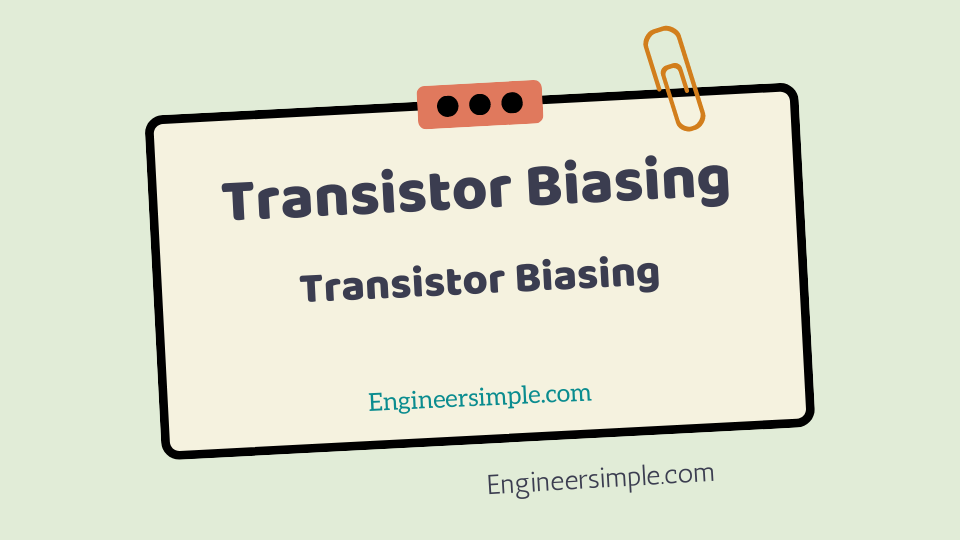Transistor Biasing - Engineer Simple

#In the transistor amplifier circuit drawn so far biasing was done with the aid of a battery
V BB which was separate from the battery V CC used in the output circuit.

However, in the
interest of simplicity and economy, it is desirable that transistor circuit should have a
single source of supply – the one in the output circuit (i.e V CC ).The following are the most commonly use methods of obtaining transistor biasing from one source of supply
(i.e. V CC ):
(i) Base resistor method
(ii) Emitter bias method
(iii) Biasing with collector-feedback resistor
(iv) Voltage-divider bias
In all these methods, the same basic principle is employ i.e. require value of base
current (and hence I C ) is obtain from V CC in the zero signal conditions.

The value of collector load R C is select keeping in view that V CE should not fall below 0.5 V for
germanium transistors and 1 V for silicon transistors.
For example, if = 100 and the zero signal collector current I C is to set at 1mA,
then I B is make equal to I C / = 1/100 = 10 A. Thus, the biasing network should be so
designed that a base current of 10 A flows in the zero signal conditions.